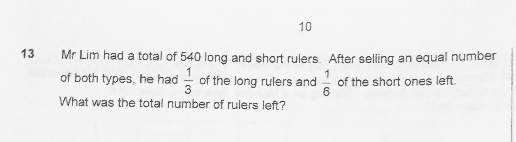# QuestionAnyone help me with attached question

long rulers ——- 3u
short rulers ——- 6p

3u + 6p ——- 540
3u – 1u = 2u
6p – 1p = 5p
2u ——- 5p
1u ——- 5p/2 = 2.5p
3 x 2.5p + 6p = 13.5p ——- 540
1p ——- 540/13.5 = 40 (short rulers left)
2.5 x 40 = 100 (long rulers left)
100 + 40 = 140

Ans : 140 rulers

0 Replies 1 Like ✔Accepted Answer

Number of long rulers sold is 2 parts out of  3 parts total of long rulers, and number of short rulers sold are 5 parts out of 6 parts total of short rulers.

Since the number of short and long rulers sold is the same, we will use a common unit to represent them. A convenient number would be 10u, since 10 is the lcm of the 2 parts of long and 5 parts of short ruler sold.

Long rulers sold = 10u, which is 2/3.

Therefore total of long rulers is 15u, i.e 3/3.

Short rulers sold = 10u, which is 5/6.

There total of short rulers = 12u, i.e 6/6.

15u + 12u = 540

u = 540/27 = 20

Long rulers left = 5u

Short ruler left = 2u

Total rulers left = 7u = 140#

Same concept just fractional presentation below:

same no. of rulers sold,

2/3 L = 5/6 S

make numerator same,

10/15 L = 10/12 S

Total units in denominator,

15 units Long + 12 units Short = 27 units

27 units at first = 540 –> 1 unit= 20

left 5 units Long and 2 units Short

7 units = 140

0 Replies 2 Likes